# The IF Function

Whether you're looking to start using the Formula Column for the first time, or you're making your way to becoming a Formula Column expert, chances are the IF function is the place you will go. With the IF function's nearly endless useful capabilities, it's no wonder that it is the most frequently used function in the Formula Column!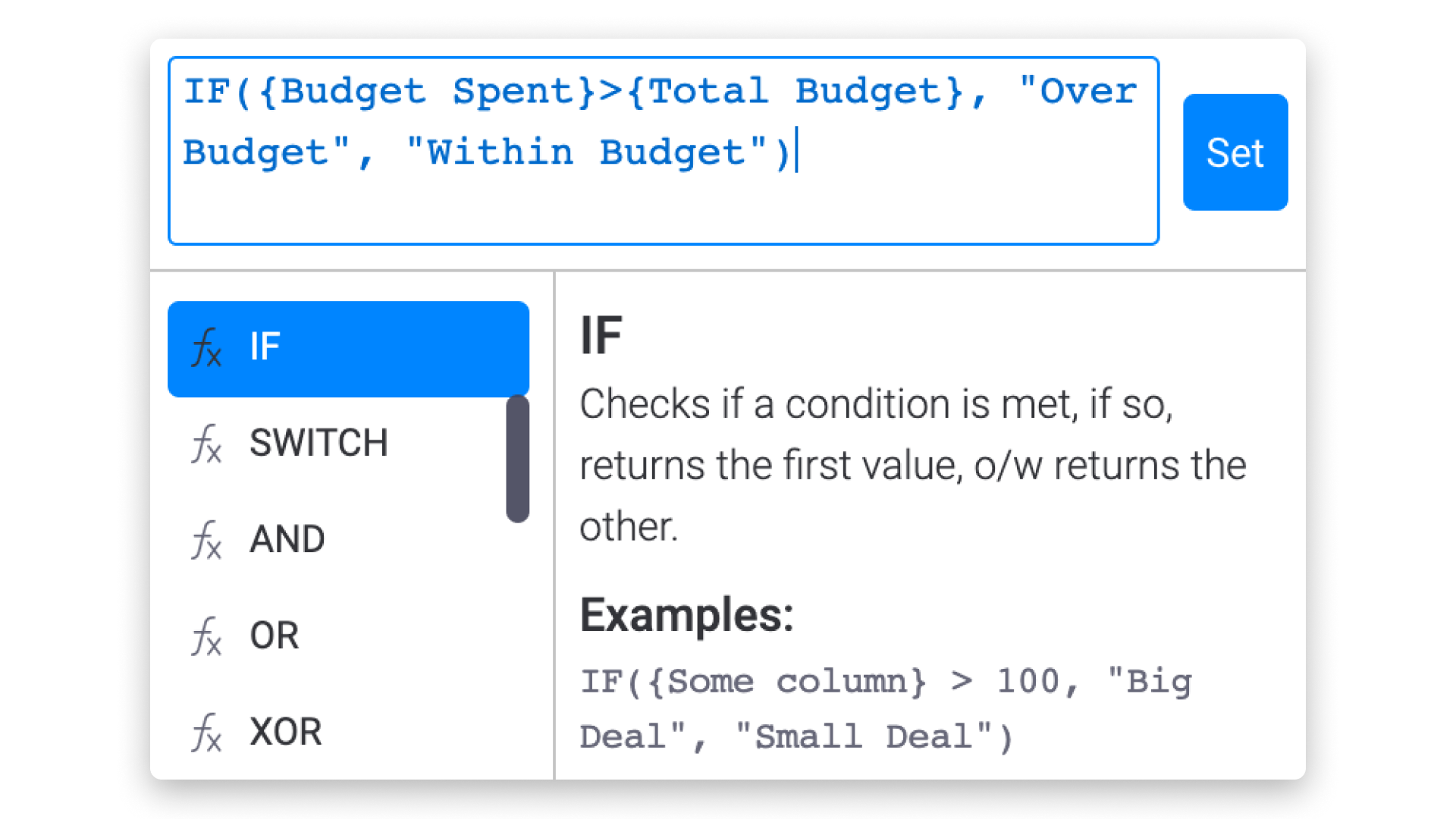In this article, we'll first learn all about the basics of the IF function and we'll explore other variations and conditions which can be added to it as well. Topics covered include:

Read on to learn all about what the IF function is, how it works, and some examples on how to use it. 🙌

# Understanding the IF Function

If you're new to the formula world, you may be wondering "What is the IF function?" Well, in essence, the IF Function is a type of formula which is used to create a conditional statement and it will return, or output, a value depending on whether the set condition is met or not.

In its simplest form, an IF Function formula is structured as follows:

1. First, the condition (ex: `IF({Status}="Done"`)
2. Then, the value returned if the condition is met (ex: `"YAY!"`).
3. Finally, the value returned if the condition is not met (ex: `"Not yet...")`)

When combined into one formula, it will look like so:Formula: `IF({Status}="Done","YAY!","Not yet...")`

Let's break it down!

In the example shown above, the formula means that if the status is set to "Done", then it will return the value of "YAY!". On the other hand, if the status is not set to "Done", then it will return the value of "Not yet...". Therefore, the result of this formula is conditional upon whether or not the status has the label of "Done".

This is what it will look like when placed on a board! ⬇️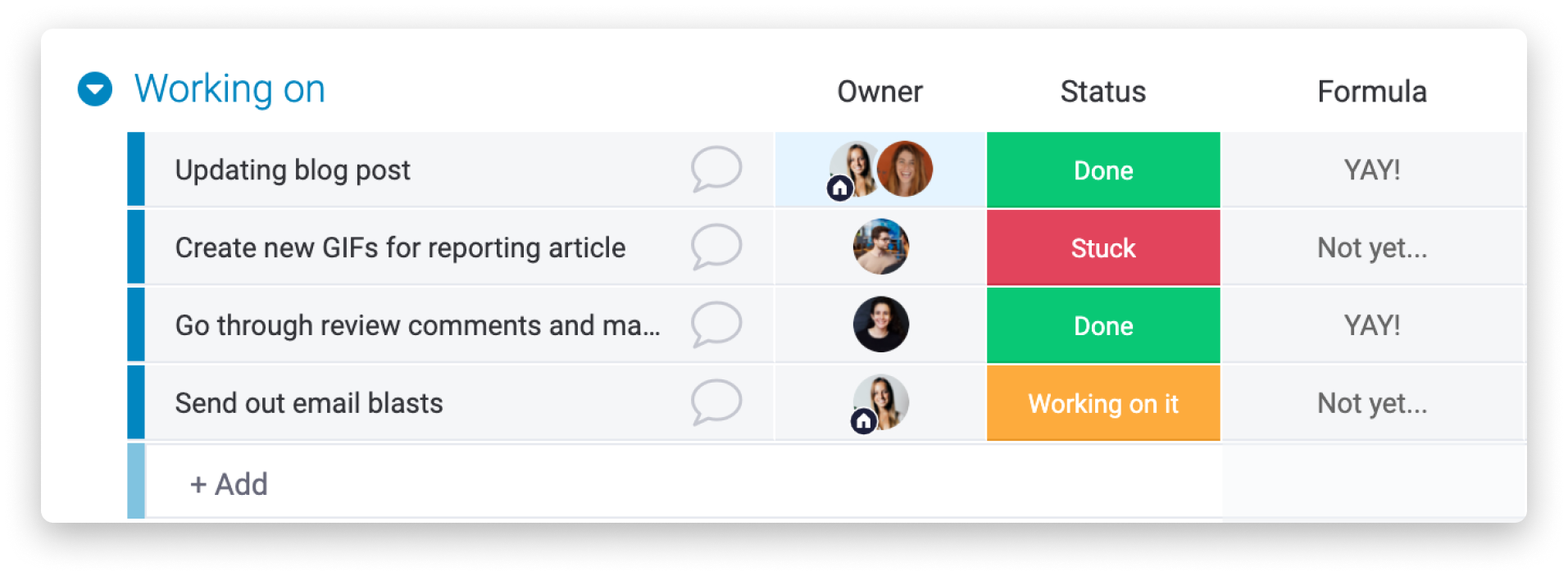Note: You must use parentheses to open and close an IF statement, as well as any other statement in a formula. Throughout this article, this will be indicated by using colored parentheses in any formula example provided.

# Simple IF function use case

Now that we understand what the IF function is, and how it is structured in its most basic form, let's look into a budget tracking use case to see how the IF function can be used in a real life example!

The board above is used to track the budget of an organization. Thanks to the Formula Column, renamed to 'Over/Under Budget', we can see at a glance how the actual amount of money spent compares to the budget set. The following formula is used here:Formula: `IF({Budgeted}<{Actual Spending},"Over Budget","Under Budget")`

With this function in place, the Formula Column will read if the "Budgeted" value is less than the "Actual Spending" value. If the "Budgeted" value is indeed less than the "Actual Spending" value, then the text "Over Budget" will be displayed. Otherwise, if this condition is not met and the "Budgeted" value is more than the "Actual Spending", then the text "Under Budget" will be shown instead.

# Using AND/OR conditions with the IF Function

When using the IF Function, you may need to specify additional conditions. This will be particularly important as you are looking to build longer and more complex calculations! For this reason, other logical functions such as AND or OR can be combined with the IF function.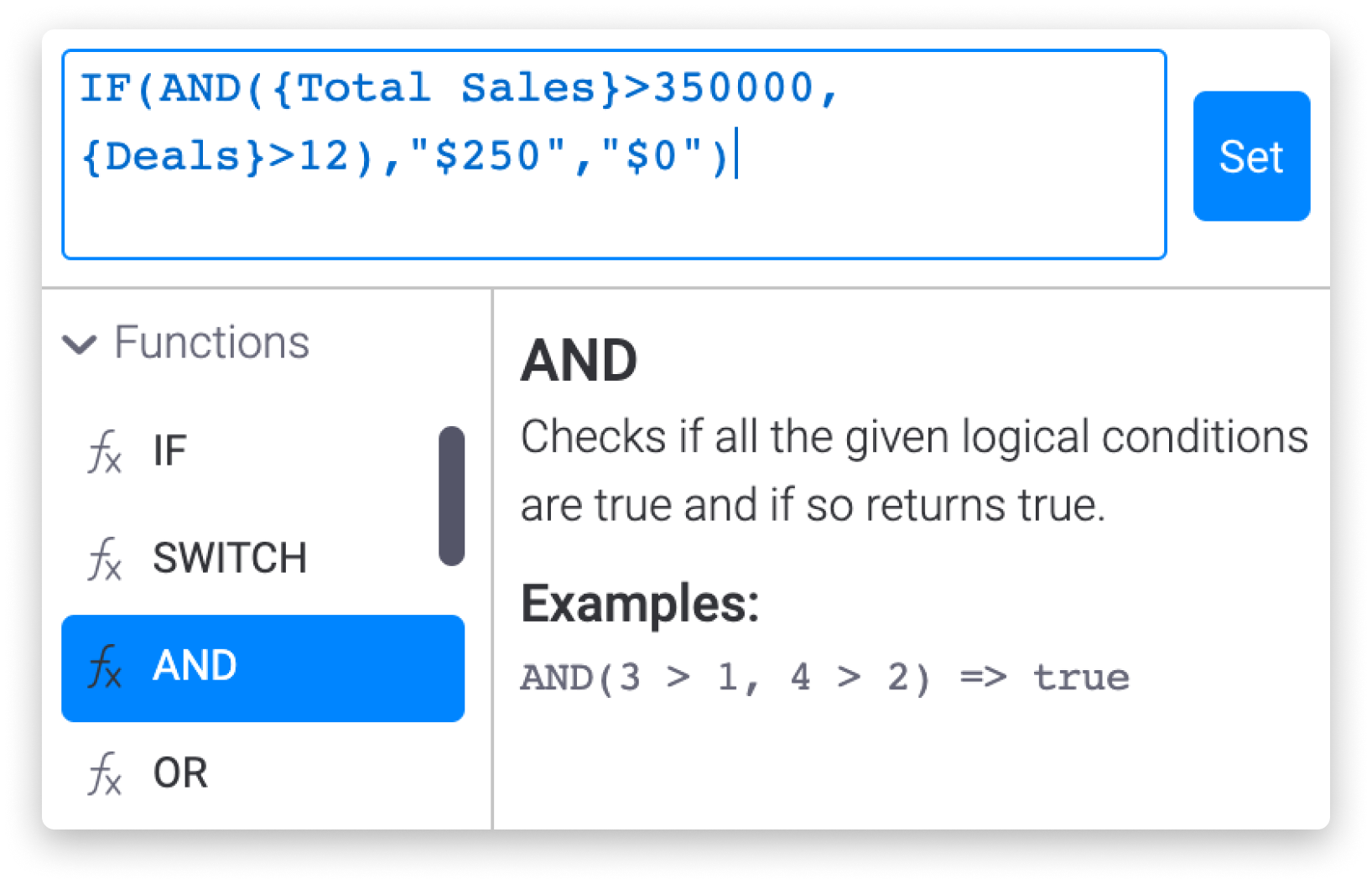As shown above, when an additional condition or function is added to an IF formula, the additional statement (i.e. `AND({Total Sales}>350000,{Deals}>12)`) will be enclosed within the initial IF statement. Let's dive further into an AND condition example, followed by an OR condition example, below to see how this works!

# AND condition

The AND function can be used within an IF formula to allow you to test for multiple conditions in order for a value to be displayed. When using this, all conditions placed must be met in order for a value to be shown. If only one or none of these conditions are met, the value placed in the formula that is defined for conditions that are not met will be shown instead.

Let's consider the below Sales Lead Management board to see an example of how the IF and AND functions can be combined to determine if a \$2,500 bonus should be received or not:

On this board, we've added a Formula Column titled 'Bonus value' to display the \$2,500 bonus amount that a Sales Rep may be eligible to receive. In order for a Sales Rep to be entitled to receive the \$2,500 bonus, the deal size must be over \$350,000 and the payment status must be marked as "Paid". The following formula can allow us to perform just that:Formula: `IF(AND({Deal size}>350000,{Payment}="Paid"),"\$2500","\$0")`

The formula example above will work as so:

• The formula will first read whether the Deal size is over 350000 and the Payment status column is marked as "Paid"
• If both of these conditions are met, the value of "\$2500" will be returned.
• Otherwise, if either of these conditions are not met, then the formula will return the value of "\$0".

Great! Thanks to the combination of the AND and IF function, our formula can help us easily determine whether a Sales Rep is qualified for a \$2,500 bonus or not! 💥

# OR condition

In a similar fashion to the AND condition, the OR function also allows you to test for multiple different conditions in order for a value to be displayed. The main difference between the two, is that for the OR condition, only one of the conditions placed must be met in order for a value to be shown.

To see this in action, let's consider the Sales Team Commissions board below! Similar to the previous use case, this board allows us to calculate a bonus rate for each of our sales reps. However, this time, the formula column (titled 'Bonus rate') considers other variables when performing this calculation.

This time, in order for a sales rep to be considered for a bonus, only one of two conditions must be met:

• Either their Total Sales amount has to be higher or equal to (>=) the Sales Goal
• or their number of accounts must be greater than or equal to (>=) the Account Goal

In order to achieve this calculation, the formula will look as so:Formula: `IF(OR({Total sales}>={Sales goal},{# accounts}>={Account goal}),{Total sales}*{Commission rate},0)`

If either of the two conditions are met (Total sales >= Sales goal or # accounts >= Account goal), then the sales rep will be qualified for a bonus which will be calculated by multiplying their Total Sales value by the Commission rate. Otherwise, if neither of these conditions are met, their Bonus rate will be \$0.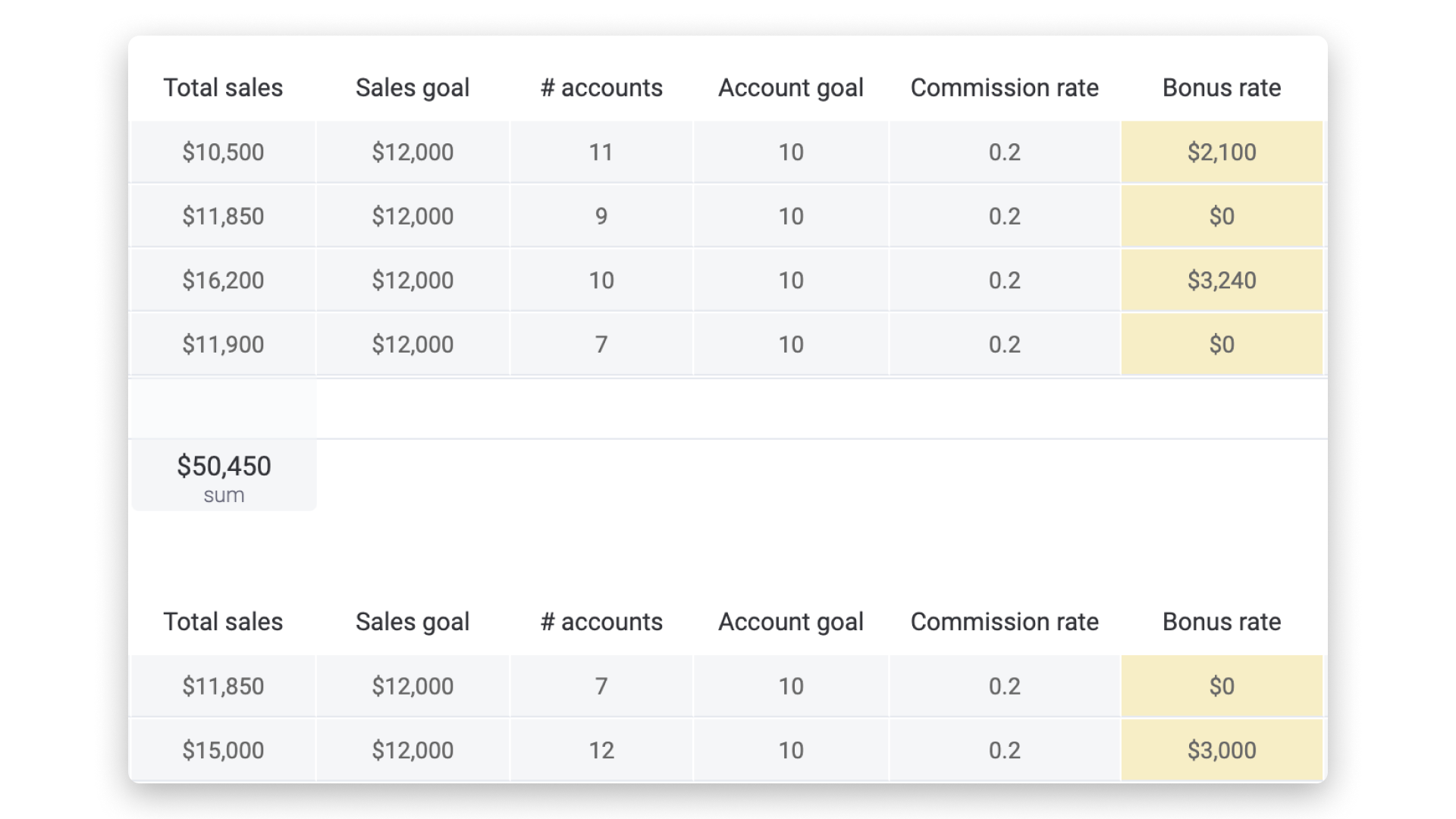# 'Nested' IF formula

The final type of IF function that will be explored here, known as a 'Nested' IF formula, works by embedding multiple IF functions insider another. This is used when you want to test for multiple different conditions and to have a specific value presented depending on which of these conditions are met.

To understand how this may work, let's consider the below board which is used to manage a T-shirt manufacturing company! The formula column, titled 'Price per unit' will be our focus for this use case.

Within this board, there are three different types of t-shirts indicated by the Status Column titled 'T-shirt Type': Ironman, Thor, and Captain America. By using a nested IF formula, we can easily and automatically display the Price per unit based off of the T-shirt type that is selected!

Before diving into this formula, it's important to note that the different T-shirt types are priced as follows:

• Thor: \$15
• Ironman: \$20
• Captain America: \$30

In order to display the price per unit, based off of the T-shirt type (status label) that is selected, we want to include three different IF functions which are nested all in one.

To create a formula that reflects this logic, we'll first test to see if the T-shirt type is "Thor", if so, the value presented is 15 (based on its cost). If this condition is not met, we move onto the next IF statement in which we test to see if the T-shirt type is "Ironman", and if so, the result will be 20. For the final option, we'll test to see if the T-shirt type is "Captain America" and if so, it will result in a value of 30. If none of these conditions are met, then 0 will be displayed.

The resulting formula will look like this:Formula: `IF({T-shirt Type}="Thor",15,IF({T-shirt Type}="Ironman",20,IF({T-shirt Type}="Captain America",30,0)))`

Notice how each IF function has its own parentheses which open each statement separately, and then they all close together in the end? This is how a nested IF function must be structured in order for it to work properly.

With this formula in place, the price per unit will automatically be displayed in response to a T-shirt type being selected! 🙌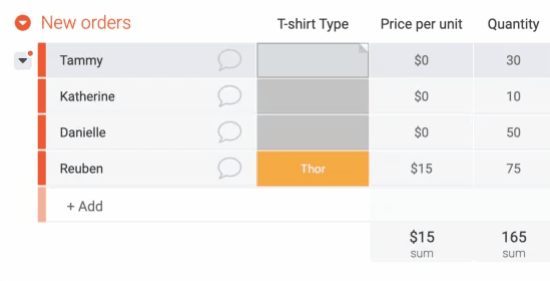Amazing! We hope that this article provided you with the foundation to begin exploring the IF function within the Formula Column. There are so many calculations that you can create with this, and you can really get creative here!Note: For more tips, tricks, and general information on how to use the Formula Column, check out this article. You can also find additional formula examples in our Formula Use Cases article.

If you have any questions, please reach out to our team by using our contact form. We're available 24/7 and happy to help!

Have more questions? Submit a request